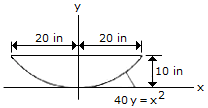# Engineering Mechanics - Moments of Inertia - Discussion

### Discussion :: Moments of Inertia - General Questions (Q.No.2)

2.Determine the inertia of the parabolic area about the x axis.

 [A]. Ix = 11,430 in.4 [B]. Ix = 32,800 in.4 [C]. Ix = 13,330 in.4 [D]. Ix = 21,300 in.4

Explanation:

No answer description available for this question.

 Dhinesh said: (May 26, 2011) Give answer descripition.

 G.Vasu From Iiit(Rkvalley) said: (Mar 6, 2012) dIx=y^2.dA where dA=x.dy 40.y=x^2 =>x=square root of(40.y) then, susbstitute the above values in main equation dIx=y^2.over root of(40.y).dy then take integral on both side from 0 to 10. then you get Ix=11,430 in^4.

 Aksharkumar said: (Oct 28, 2013) Determine the moment of inertia for section about horizontal and vertical centroidal 120.

 Vio said: (Sep 25, 2014) dIx = y^2.dA. Where dA = x.dy. 40.y = x^2. => x=square root of(40.y). Then, susbstitute the above values in main equation, dIx = y^2.over root of(40.y).dy. Then take integral on both side from 0 to 10. Then you get Ix=11,430 in^4.